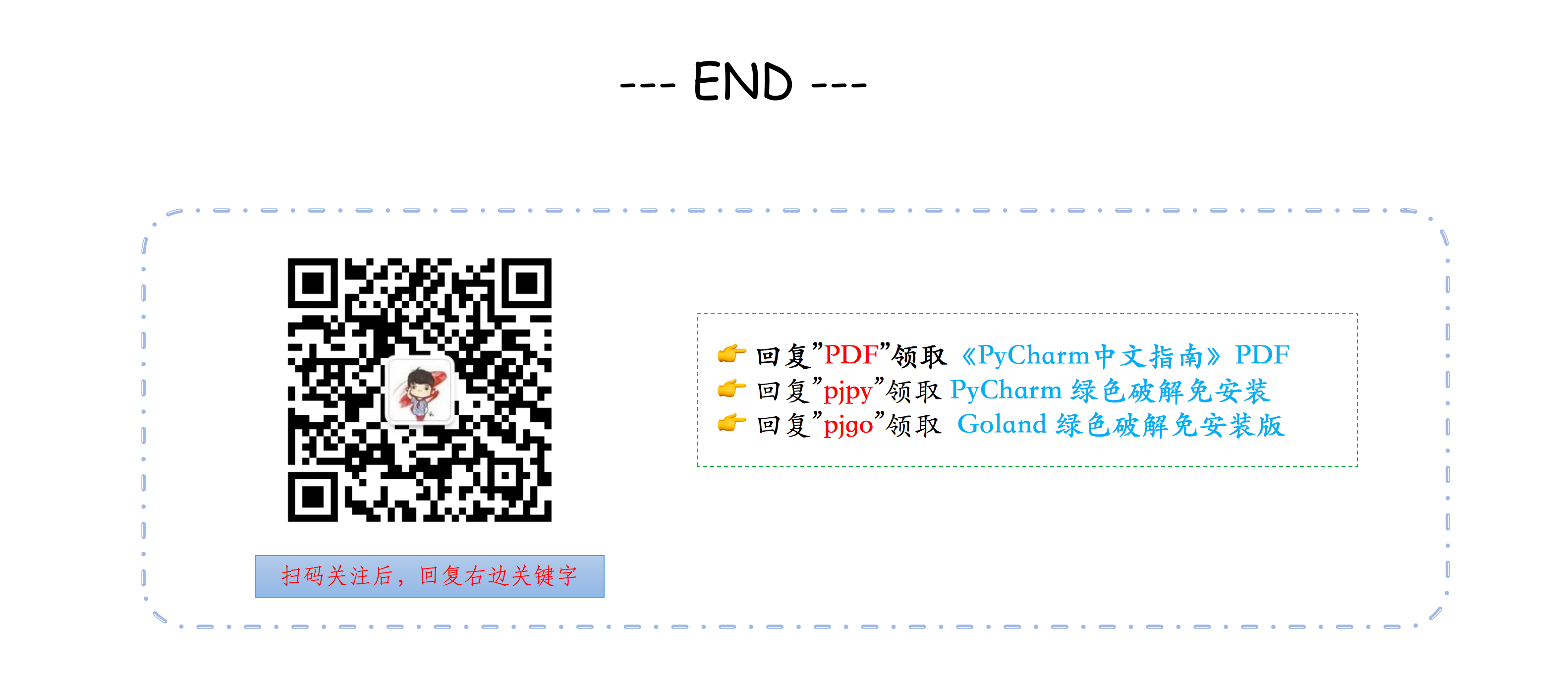# 记录几个 Python 字典中的冷门操作

## 01. 键映射多个值

d = {
'a' : [1, 2],
'b' : 
}
e = {
'a' : {1, 2},
'b' : {3}
}


d=dict()
d.setdefault('a',[]).append(1)
d.setdefault('a',[]).append(2)
d.setdefault('b',[]).append(3)

e=set()
e.setdefault('a',{}).add(1)
e.setdefault('a',{}).add(2)
e.setdefault('b',{}).add(3)


from collections import defaultdict

d = defaultdict(list)
d['a'].append(1)
d['a'].append(2)
d['b'].append(4)

d = defaultdict(set)
d['a'].add(1)
d['a'].add(2)
d['b'].add(4)


## 02. 有顺序的字典

from collections import OrderedDict

d = OrderedDict()
d['foo'] = 1
d['bar'] = 2
d['spam'] = 3
d['grok'] = 4

for k in d:
print(k, d[k])

import json
json.dumps(d)


('foo', 1)
('bar', 2)
('spam', 3)
('grok', 4)

'{"foo": 1, "bar": 2, "spam": 3, "grok": 4}'


OrderedDict 内部维护着一个根据键插入顺序排序的双向链表。每次当一个新的元素插入进来的时候， 它会被放到链表的尾部。对于一个已经存在的键的重复赋值不会改变键的顺序。

## 03. 键值的反转

>>> keys = ['name', 'age', 'food']
>>> values = ['Monty', 42, 'spam']
>>>
>>> zip(keys, values)
[('name', 'Monty'), ('age', 42), ('food', 'spam')]
>>>
>>> dict(zip(keys, values))
{'food': 'spam', 'age': 42, 'name': 'Monty'}


>>> d={'food': 'spam', 'age': 42, 'name': 'Monty'}
>>> zip(d.values(), d.keys())
[('spam', 'food'), (42, 'age'), ('Monty', 'name')]
>>>
>>> dict(zip(d.values(), d.keys()))
{42: 'age', 'Monty': 'name', 'spam': 'food'}


## 04. 求最大值对应的键

>>>prices={"Apple": 6, "Mango": 7, "Pear":3}


>>> max(zip(prices.values(), prices.keys()))
(7, 'Mango')


>>> max(prices, key=lambda k:prices[k])
'Mango'